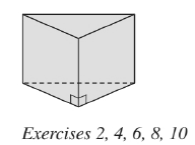Chapter 9.1, Problem 6EElementary Geometry For College St...

7th Edition
Alexander + 2 others
ISBN: 9781337614085

Solutions

Chapter
SectionElementary Geometry For College St...

7th Edition
Alexander + 2 others
ISBN: 9781337614085
Textbook Problem

If each edge of the triangular prism in Exercise 2is measured in inches, what unit is used to measure its a ) lateral area? b ) volume?To determine

a)

To find:

The unit used to measure lateral area of the triangular prism when each of the edge is measured is inches.

Explanation

Calculation:

Given,

The lateral area of the triangular prism with base perimeter ‘P’ and altitude ‘h

L=

To determine

b)

To find:

The unit used to measure volume of the triangular prism when each of the edge is measured is inches.

Still sussing out bartleby?

Check out a sample textbook solution.

See a sample solution

The Solution to Your Study Problems

Bartleby provides explanations to thousands of textbook problems written by our experts, many with advanced degrees!

Get Started

Find more solutions based on key concepts